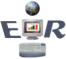Excel Basics

 General InformationFrom the Start Menu, choose Programs then choose Microsoft Excel.  Or Double click on the Excel Icon if it is located on your desktop. Excel is a spreadsheet application, general used for calculation.  It normally opens with 3 spreadsheets available.  Sheets can be added, removed or relocated as you see fit. Click here to study the screen in more detail. Each sheet has 256 columns and 65,536 rows.  Yes, that is an absurd amount, but you have to remember in the old days (back in the late 70's) the program only had the availability of one sheet and working on major project was a lot more difficult as you had to spread it out over the sheet. As with all programs there are visual cues as to your location on the sheet.  Unlike a word processing program, the cursor is only visible within a cell or on the formula bar.   The first indicator is the rectangular box.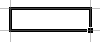Your location is also shown in the name box field.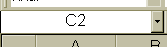Your last indicator is the column and row identifiers are bolded.

Insert text
 On the new document the cursor will be located at the beginning of the document, cell A1.  Start typing the text.

Delete text
 When in a cell, if the text you want to delete is to the ... ... right of the cursor Press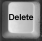... left of the cursor Press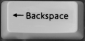Enter formulas

All formulas begin with an equal sign (=)
Mathematical symbols
 Multiplication (*) the asterisk key located either on top of the number 8 key or on the numeric keypad. Division (/) the regular slash key, usually located on the right side of the keyboard or on the numeric keypad Addition (+) The plus key, located either over the = sign, on the upper right side of the keyboard or the numeric keypad Subtraction (-) The dash key, located next to the 0 on the upper row of the keyboard, or the upper right of the numeric keypad
When you begin a formula you should be in the cell that you want the result to appear in.  For example, let's say you want to figure out some sort of tax on sales.  In a situation like that, you would need a minimum or three columns.  One to hold the sale amount, the second to input the tax rate and the third for the result.

Generally, the first row of a spreadsheet is saved for the titles of the columns.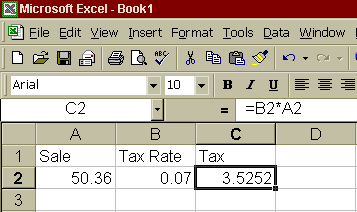Following are some examples of common formulas.
 =A1*B1 The value in cell A1 is multiplied by the contents of cell B1 =Sum(A1:F1) Add the values of cells from A1 to F1 =Sum(A1,C1,F1) Add the values of on the cells A1, C1 and F1 =Sum(A1:F1,H1,L1) Add the values of cells from A1 to F1, plus the values of H1 and L1 =A1/B1 Divide the amount in A1 by the value of B1 =A1+((B1*C1)-(D1/E1)) Add the value of A1 to the total of (B1*C1)-(D1/E1) =IF(B1>0,"Yes","No") If the value of B1 is greater than 0, insert the word "Yes" otherwise, insert "No"
It should be noted that when formulas are copied, the referential locations will be increased by the incremental row or column you move.

For example,
If you have a formula in column C1 that reads    =A1*B1 and copy it down one row,
the formula on row two (C2) will read =A2*B2.

Conversely, if you should copy this formula right the new formula will read =B1*C1

Should you require an absolute cell reference, place a \$ in front of the column or row you don’t want changed when copied.
 The original formula When copied The resulting formula would be =A1*C\$1 down one row =A2*C\$1. right one column =B1*D\$1 =A1*\$C1 down one row =A2*\$C2. right one column =B1*\$C1 =A1*\$C\$1 down one row =A2*\$C\$1. right one column =B1*\$C\$1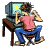Thanks for visiting  ER  Computer  Services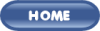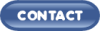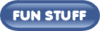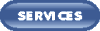These links will lead you into our framed website.  It is best viewed at a screen resolution of 1024 x 768 dpi, however, 800 x 600 dpi will also work.   If your screen cannot handle this, please navigate using our Site Map page and your browser.